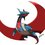# s=s... again?

view my set

we remember that $\sum_{n=1}^\infty\dfrac{Ch(n)}{n^s}=-2\zeta(2s)+\zeta(s)$ consider $Cs(n)=(Ch*1)(n)=\sum_{d|n}Ch(d)$ so, if d is a perfect square it is -1, 1 otherwise. if there are k perfect square divisors, there are $\tau(n)-k$ non-square divisor. add them and it is $\tau(n)-2k$.

let k be a function of n. It is multiplicative. So we can compute for prime powers $k(p^a)=\sum_{d^2|p^a} d^{0}=1^0+(p^2)^0+(p^4)^0+.... \left(p^{2\lfloor \dfrac{a}{2}\rfloor}\right)^0=\lfloor \dfrac{a}{2}\rfloor+1$ we have $\sum_{n=1}^\infty\dfrac{Ch(n)}{n^s}\sum_{n=1}^\infty\dfrac{1}{n^s}=\sum_{n=1}^\infty\dfrac{\tau(n)}{n^s}-2\sum_{n=1}^\infty\dfrac{k(n)}{n^s}$ $-2\zeta(s)\zeta(2s)+\zeta^2(s)=\zeta^2(s)-2\sum_{n=1}^\infty\dfrac{k(n)}{n^s}$ $\sum_{n=1}^\infty\dfrac{k(n)}{n^s}=\zeta(s)\zeta(2s)$ Lets try to compute the summation using the fact it is multpicative $\prod_{p=prime} \sum_{a≥0}\dfrac{k(p^a)}{p^{as}}=\prod_{p=prime} \sum_{a≥0}\dfrac{\lfloor \dfrac{a}{2}\rfloor+1}{p^{as}}=\prod_{p=prime} \dfrac{p^{3s}}{(p^s-1)^2(p^s+1)}\\=\prod_p \dfrac{1}{(1-p^{-s})^2}\dfrac{1-p^ {-s}}{1-p^{-2s}}=\zeta^2(s)\dfrac{\zeta(2s)}{\zeta(s)}=\zeta(s)\zeta(2s)$ so we get $\boxed{\large{s=s}}$ again!!!Note by Aareyan Manzoor
5 years, 5 months ago

This discussion board is a place to discuss our Daily Challenges and the math and science related to those challenges. Explanations are more than just a solution — they should explain the steps and thinking strategies that you used to obtain the solution. Comments should further the discussion of math and science.

When posting on Brilliant:

• Use the emojis to react to an explanation, whether you're congratulating a job well done , or just really confused .
• Ask specific questions about the challenge or the steps in somebody's explanation. Well-posed questions can add a lot to the discussion, but posting "I don't understand!" doesn't help anyone.
• Try to contribute something new to the discussion, whether it is an extension, generalization or other idea related to the challenge.

MarkdownAppears as
*italics* or _italics_ italics
**bold** or __bold__ bold
- bulleted- list
• bulleted
• list
1. numbered2. list
1. numbered
2. list
Note: you must add a full line of space before and after lists for them to show up correctly
paragraph 1paragraph 2

paragraph 1

paragraph 2

[example link](https://brilliant.org)example link
> This is a quote
This is a quote
    # I indented these lines
# 4 spaces, and now they show
# up as a code block.

print "hello world"
# I indented these lines
# 4 spaces, and now they show
# up as a code block.

print "hello world"
MathAppears as
Remember to wrap math in $$ ... $$ or $ ... $ to ensure proper formatting.
2 \times 3 $2 \times 3$
2^{34} $2^{34}$
a_{i-1} $a_{i-1}$
\frac{2}{3} $\frac{2}{3}$
\sqrt{2} $\sqrt{2}$
\sum_{i=1}^3 $\sum_{i=1}^3$
\sin \theta $\sin \theta$
\boxed{123} $\boxed{123}$

Sort by:

The combo part was not found by me but @Andrew Ellinor so he gets the credit for that.

He was not sure wether or not he was correct but this proves that $k(n)=\prod_{i=1}^{\omega(n)} (\lfloor \dfrac{k_i}{2}\rfloor +1)$ if $n=p_1^{k_1}p_2^{k_2}.....p_{\omega(n)}^{k_{\omega(n)}}$

- 5 years, 5 months ago

Well he is correct.

- 5 years, 5 months ago